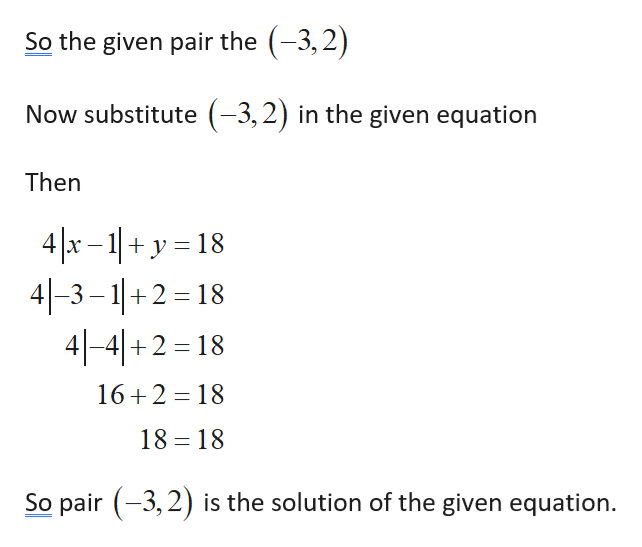# Determine if the given ordered pair is a solution to the equation 4|x − 1| + y= 18.a.(−3, 2)b.(5, −2)

Question
9 views

Determine if the given ordered pair is a solution to the equation 4|x − 1| + y= 18.

a.(−3, 2)

b.(5, −2)

check_circle

Step 1

Consider the given equation

Step 2

Subpart(a...help_outlineImage TranscriptioncloseSo the given pair the (-3,2) Now substitute (-3,2) in the given equation Then 4|x - 1| + y = 18 4|-3 – 1|+2 = 18 4|-4|+2 = 18 16+2 = 18 18 = 18 So pair (-3, 2) is the solution of the given equation. fullscreen

### Want to see the full answer?

See Solution

#### Want to see this answer and more?

Solutions are written by subject experts who are available 24/7. Questions are typically answered within 1 hour.*

See Solution
*Response times may vary by subject and question.
Tagged in

### Equations and In-equations